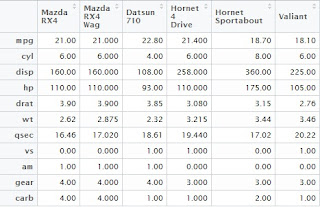## Pages

###R Tutorial 10.0

Let's us understand how to transpose data in R.

There are two methods to do so. 1. Simply use function "t" for flat transposing and 2. using reshape package for customized transposing.

For much details, read the complete article and examples provided.

### How to transpose data in R

Just use a "t" to do so !

#Lets prepare a data and then try to transpose it

rm(list = ls())   # Let's clearn work space

Name = c("A","B","C","D","E","F","G","H")
Age = c(22,23,24,20,19,24,22,23)
Gender= c("M","F","M","F","M","F","M","F")
Earning= c(800,700,500,1000,1100,800,700,600)
Expense = c(100,110,120,110,130,90,100,80)
Data_1 = data.frame(Name,Age,Gender, Earning, Expense)
rm(Name,Age,Gender,Earning,Expense)

Data_2 = t(Data_1)Please click to enlargeData_mt

We can also try the transpose command on an inbuilt dataset of R.

# Including head function to keep only few (6) observations for sample

### User Reshape package for reshaping the data

Let's try to learn two functions melt() and cast() which are very useful for reshaping the datasets.We will create a subset data from Data_1 (created above), with only 4 columns : Age, Gender, Earning and Expense.

Data_10 = Data_1[ , 2:5]

The melt function basically creates all the unique combinations of id variables and then rest of the variables are transposed. We need to invoke the library reshape for using melt function.

library(reshape)
New_Data <- melt(Data_10, id=c("Age","Gender"))

#  Now on this molten data (New_Data), we can use cast function.
Mean_values <- cast(New_data, Gender~variable, mean)

Enjoy reading our other articles and stay tuned with us.

Kindly do provide your feedback in the 'Comments' Section and share as much as possible.